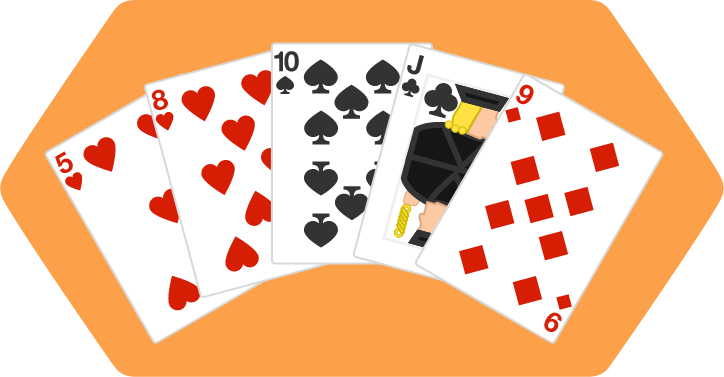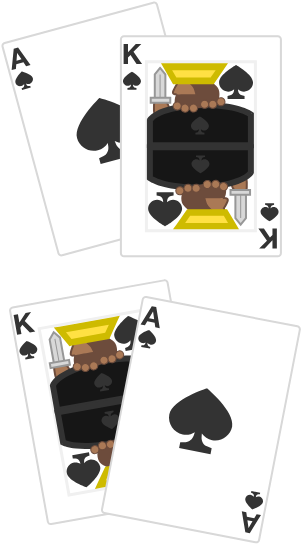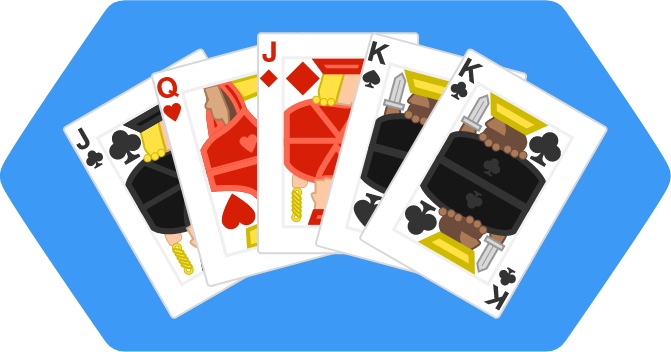## All Faces

How many possible $5$-card hands of poker are there?The simple answer to this question is — there are a lot. But to give a precise answer to this question, let's start with something much less complicated: how many possible hands of $2$ cards are there?

Suppose we drew $1$ card from a shuffled standard deck. There would be $52$ possibilities for this card. Then, we draw another card from the same deck. After the first card is drawn, the deck only contains $51$ cards, so there are $51$ possibilities for the second card.

This means that there are $52 \times 51=2652$ ways to draw $2$ cards from a standard deck. Does this mean that the number of possible hands of $2$ cards is $2652?$

As it turns out, we're leaving an important piece of information out of this. Each possible hand of $2$ cards contains $2$ different permutations.You can draw these cards in two different orders, but they both represent the same hand of two cards.

In other words, there are always $2$ different ways to draw the same hand. Since we know that there are $2$ permutations for each hand, we can simply take $2652 \div 2 = 1326,$ and this is the number of possible hands of $2$ cards.

In mathematical terms, we've just computed the number of combinations of $2$ cards out of the $52$ in a standard deck. In mathematical notation, it looks like this:

$_{52}\text{C}_{2}=\binom{52}{2} = 1326.$

The two different notations on the left mean the same thing, but the binomial coefficient $\binom{\cdot}{\cdot}$ is more common when dealing with poker probabilities.

The number of possible $5$-card hands in poker will be expressed as $\binom{52}{5},$ and to calculate it, we'll follow a similar line of thinking. First, we'll compute the number of ways to draw $5$ cards from a deck.

There are $52$ possibilities for the first card, then $51$ possibilities for the second card, and so on. This means there are $52 \times 51 \times 50 \times 49 \times 48$ ways to draw $5$ cards from the deck.

Then, we need to account for all the permutations of the same hand of $5$ cards. Fortunately, this is a very similar calculation: There are $5$ possible ways to choose the first card, then $4$ ways to choose the second card, and so on. In total, there are $5 \times 4 \times 3 \times 2 \times 1$ permutations of $5$ cards.

Each hand has the same number of permutations, so the number of possible $5$-card poker hands is

\begin{aligned} \binom{52}{5} &= \frac{52 \times 51 \times 50 \times 49 \times 48}{5 \times 4 \times 3 \times 2 \times 1} \\ &= 2598960. \end{aligned}

This is a staggering amount. If you stacked the possible $5$-card hands on top of one another, the stack would tower over the tallest building in the world (the Burj Khalifa in Dubai) and would rival the height of mountains.

You can use the same method above to compute the number of specific types of hands in poker, and then find the probability of those hands. For example, let's suppose we wanted to find the probability of drawing $5$ cards of the same suit when drawing $5$ cards from a shuffled deck.

There are $4$ possible suits, and each suit has $13$ cards. To form this type of hand, we want $5$ of those cards, so the probability is

\begin{aligned} \frac{4\times \binom{13}{5}}{\binom{52}{5}} &= \frac{5148}{2598960} \\ &\approx 0.00198. \end{aligned}

# Today's Challenge

The Jack, Queen, and King are the face cards of a standard poker deck.This is an example of the type of hand described below.

If you draw $5$ cards from a shuffled poker deck, what is the approximate probability that all $5$ cards are face cards?

×